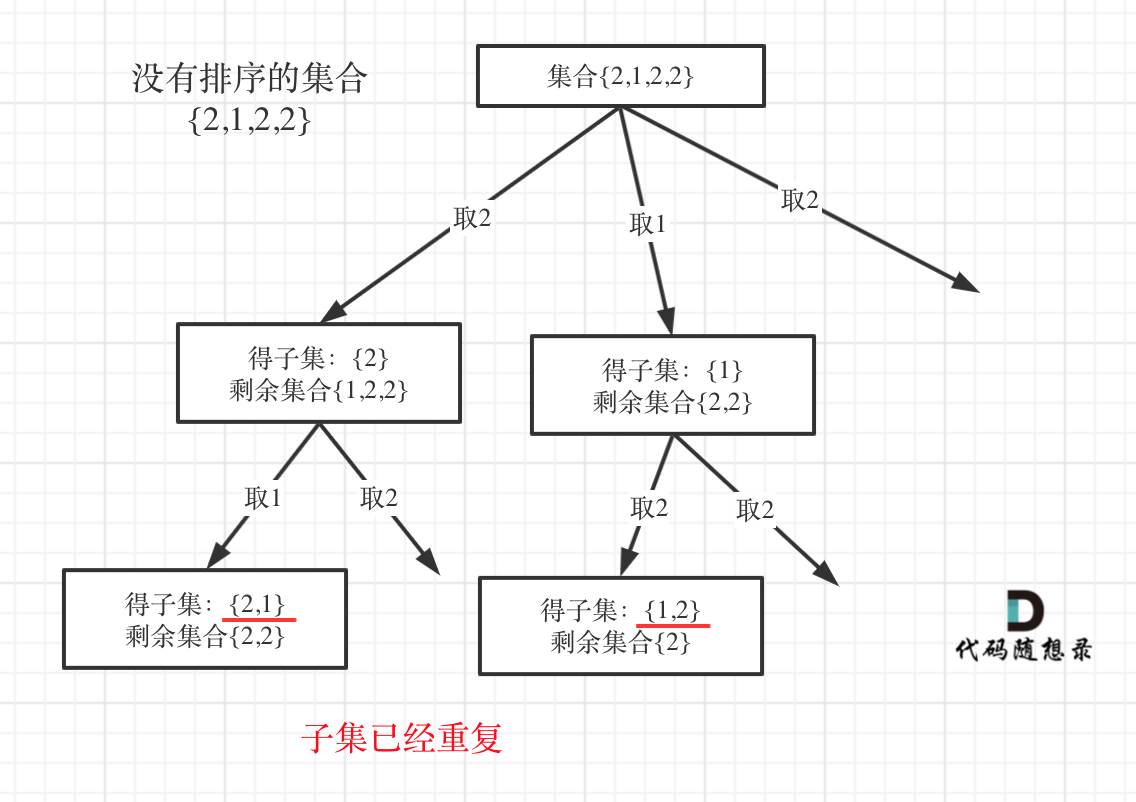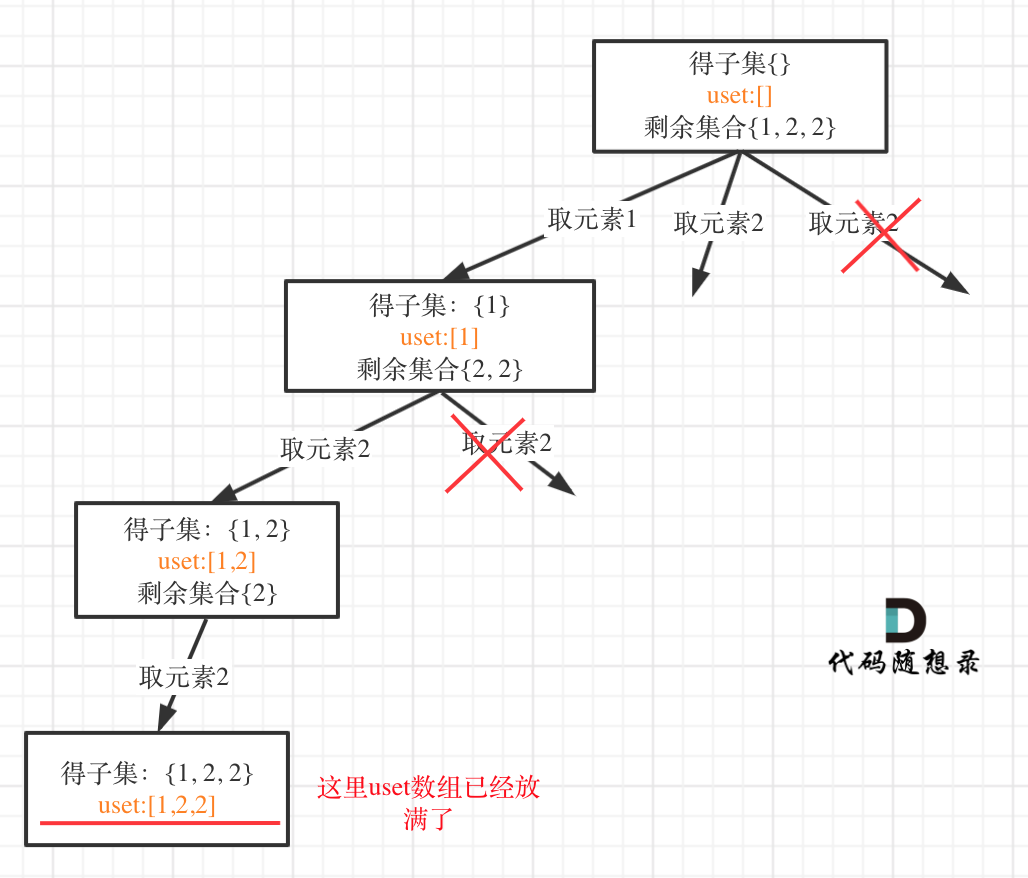# # 回溯算法去重问题的另一种写法## # 90.子集II

used数组去重版本： 回溯算法：求子集问题（二） (opens new window)

``````class Solution {
private:
vector<vector<int>> result;
vector<int> path;
void backtracking(vector<int>& nums, int startIndex, vector<bool>& used) {
result.push_back(path);
unordered_set<int> uset; // 定义set对同一节点下的本层去重
for (int i = startIndex; i < nums.size(); i++) {
if (uset.find(nums[i]) != uset.end()) { // 如果发现出现过就pass
continue;
}
uset.insert(nums[i]); // set跟新元素
path.push_back(nums[i]);
backtracking(nums, i + 1, used);
path.pop_back();
}
}

public:
vector<vector<int>> subsetsWithDup(vector<int>& nums) {
result.clear();
path.clear();
vector<bool> used(nums.size(), false);
sort(nums.begin(), nums.end()); // 去重需要排序
backtracking(nums, 0, used);
return result;
}
};

``````
1
2
3
4
5
6
7
8
9
10
11
12
13
14
15
16
17
18
19
20
21
22
23
24
25
26
27
28
29

### # 错误写法一

``````class Solution {
private:
vector<vector<int>> result;
vector<int> path;
unordered_set<int> uset; // 把uset定义放到类成员位置
void backtracking(vector<int>& nums, int startIndex, vector<bool>& used) {
result.push_back(path);

for (int i = startIndex; i < nums.size(); i++) {
if (uset.find(nums[i]) != uset.end()) {
continue;
}
uset.insert(nums[i]);   // 递归之前insert
path.push_back(nums[i]);
backtracking(nums, i + 1, used);
path.pop_back();
uset.erase(nums[i]);    // 回溯再erase
}
}

``````
1
2
3
4
5
6
7
8
9
10
11
12
13
14
15
16
17
18
19
20### # 错误写法二

``````class Solution {
private:
vector<vector<int>> result;
vector<int> path;
unordered_set<int> uset; // 把uset定义放到类成员位置
void backtracking(vector<int>& nums, int startIndex, vector<bool>& used) {
result.push_back(path);
uset.clear(); // 到每一层的时候，清空uset
for (int i = startIndex; i < nums.size(); i++) {
if (uset.find(nums[i]) != uset.end()) {
continue;
}
uset.insert(nums[i]); // set记录元素
path.push_back(nums[i]);
backtracking(nums, i + 1, used);
path.pop_back();
}
}
``````
1
2
3
4
5
6
7
8
9
10
11
12
13
14
15
16
17
18

uset已经是全局变量，本层的uset记录了一个元素，然后进入下一层之后这个uset（和上一层是同一个uset）就被清空了，也就是说，层与层之间的uset是同一个，那么就会相互影响。

## # 40. 组合总和 II

``````class Solution {
private:
vector<vector<int>> result;
vector<int> path;
void backtracking(vector<int>& candidates, int target, int sum, int startIndex) {
if (sum == target) {
result.push_back(path);
return;
}
unordered_set<int> uset; // 控制某一节点下的同一层元素不能重复
for (int i = startIndex; i < candidates.size() && sum + candidates[i] <= target; i++) {
if (uset.find(candidates[i]) != uset.end()) {
continue;
}
uset.insert(candidates[i]); // 记录元素
sum += candidates[i];
path.push_back(candidates[i]);
backtracking(candidates, target, sum, i + 1);
sum -= candidates[i];
path.pop_back();
}
}

public:
vector<vector<int>> combinationSum2(vector<int>& candidates, int target) {
path.clear();
result.clear();
sort(candidates.begin(), candidates.end());
backtracking(candidates, target, 0, 0);
return result;
}
};
``````
1
2
3
4
5
6
7
8
9
10
11
12
13
14
15
16
17
18
19
20
21
22
23
24
25
26
27
28
29
30
31
32

## # 47. 全排列 II

``````class Solution {
private:
vector<vector<int>> result;
vector<int> path;
void backtracking (vector<int>& nums, vector<bool>& used) {
if (path.size() == nums.size()) {
result.push_back(path);
return;
}
unordered_set<int> uset; // 控制某一节点下的同一层元素不能重复
for (int i = 0; i < nums.size(); i++) {
if (uset.find(nums[i]) != uset.end()) {
continue;
}
if (used[i] == false) {
uset.insert(nums[i]); // 记录元素
used[i] = true;
path.push_back(nums[i]);
backtracking(nums, used);
path.pop_back();
used[i] = false;
}
}
}
public:
vector<vector<int>> permuteUnique(vector<int>& nums) {
result.clear();
path.clear();
sort(nums.begin(), nums.end()); // 排序
vector<bool> used(nums.size(), false);
backtracking(nums, used);
return result;
}
};
``````
1
2
3
4
5
6
7
8
9
10
11
12
13
14
15
16
17
18
19
20
21
22
23
24
25
26
27
28
29
30
31
32
33
34

## # 两种写法的性能分析

used数组可是全局变量，每层与每层之间公用一个used数组，所以空间复杂度是O(n + n)，最终空间复杂度还是O(n)。

Java：

Python：

Go：